# Expression of a variable from the formula + ratio - math problems

#### Number of problems found: 129

• A perimeter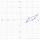A perimeter of a parallelogram is 2.8 meters. The length of one of its sides is equal to one-seventh of the entire perimeter. Find lengths of the sides of the parallelogram.
• The ratio 4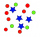The ratio of two number is 5:4 if 40% of the first number is 12, what will be 50% of the second number?
• Two fifth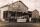Two fifth of the worker in a factory are men. The rest are women. If there 28 men . how many more women than men are there?
• Boys and girls 8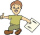In a class of 35 boys and girls that has a ratio of 2:5, 5 boys are absent and 5 boys are present. How many students are present?
• Centre of the hypotenuseFor the interior angles of the triangle ABC, alpha beta and gamma are in a ratio of 1: 2: 3. The longest side of the AB triangle is 30 cm long. Calculate the perimeter of the triangle CBS if S is the center of the side AB.
• Center of gravity and medianIn the isosceles triangle ABC, the center of gravity T is 2 cm from the base AB. The median parallel to the AB side measures 4 cm. What is the area of the ABC triangle?
• TranslateTranslate the following mathematical statement into an algebraic expression or equation. 1. Nine less that the quotient of a number and 3 2. The ratio of 3 and the sum of 4 and an unknown number 3. Eighty is the product of 4 and g 4. The sum of x and 18 i
• CalculateCalculate the area of triangle ABC, if given by alpha = 49°, beta = 31°, and the height on the c side is 9cm.
• The truncatedThe truncated rotating cone has bases with radii r1 = 8 cm, r2 = 4 cm and height v = 5 cm. What is the volume of the cone from which the truncated cone originated?
• The diamondThe diamond has an area S = 120 cm2, the ratio of the length of its diagonals is e: f = 5: 12. Find the lengths of the side and the height of this diamond.
• Ratio in trapeziumThe height v and the base a, c in the trapezoid ABCD are in the ratio 1: 6: 3, its content S = 324 square cm. Peak angle B = 35 degrees. Determine the perimeter of the trapezoid
• Cuboid - ratioFind the volume of a block whose dimensions are in the ratio 2: 3: 4 and the surface is 117 dm2.
• Cuboid edges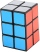The lengths of the cuboid edges are in the ratio 2: 3: 4. Find their length if you know that the surface of the cuboid is 468 m2.
• What is 10What is the 5th term, if the 8th term is 80 and common ratio r =1/2?
• If the 3If the 6th term of a GP is 4 and the 10th is 4/81, find common ratio r.
• Railway embankmentThe railway embankment section is an isosceles trapezoid, the sizes of the bases of which are in the ratio 5: 3. The arms have a length of 5 m, and the height of the embankment is 4.8 m. Calculates the size of the embankment section area.
• Vertical rodThe vertical one-meter-long rod casts a shadow 150 cm long. Calculate the height of a column whose shadow is 36 m long at the same time.
• Volume of sphereHow many times does the volume of a sphere increase if its radius increases 2 ×?
• The string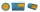They cut 113 cm from the string and divided the rest in a ratio of 5: 6.5: 8: 9.5. The longest part measured 38 cm. Find the original length of the string.
• Sphere in coneA sphere is inscribed in the cone (the intersection of their boundaries consists of a circle and one point). The ratio of the surface of the ball and the contents of the base is 4: 3. A plane passing through the axis of a cone cuts the cone in an isoscele

Do you have an exciting math question or word problem that you can't solve? Ask a question or post a math problem, and we can try to solve it.

We will send a solution to your e-mail address. Solved examples are also published here. Please enter the e-mail correctly and check whether you don't have a full mailbox.

Check out our ratio calculator. Expression of a variable from the formula - math problems. Ratio - math problems.